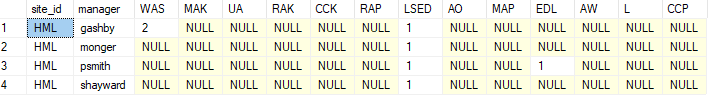troubleshooting Question

# Problem with multi-pivot query SQL serverasked on
SQLMicrosoft SQL Server
I am trying to pivot a set of terms in three different categories as follows:
``````create table test_piv(
site_id varchar(4),
Manager varchar(32),
PTR int,
PTA int,
UPS int,
ABSM int,
UCC int,
MCSID varchar(4))
``````
``````insert into test_piv values('HML','gashby',1,0,0,0,0,'WAS')
insert into test_piv values('HML','gashby',1,0,0,0,0,'WAS')
insert into test_piv values('HML','shayward',0,1,0,0,0,'LSED')
insert into test_piv values('HML','gashby',0,1,0,0,0,'LSED')
insert into test_piv values('HML','gashby',0,1,0,0,0,'LSED')
insert into test_piv values('HML','gashby',0,1,0,0,0,'LSED')
insert into test_piv values('HML','monger',0,1,0,0,0,'LSED')
insert into test_piv values('HML','psmith',0,1,0,0,0,'LSED')
insert into test_piv values('HML','psmith',0,0,1,0,0,'EDL')
``````
``````select site_id,manager,Sum([WAS]) as WAS,Sum([MAK]) As MAK,Sum([UA]) as UA ,Sum([RAK]) as RAK,Sum([CCK]) as CCK,Sum([RAP]) as RAP ,Sum([LSED]) as LSED ,Sum([AO]) as AO,Sum([MAP]) as MAP ,Sum([EDL]) as EDL,Sum([AW]) as AW ,Sum([L]) as L ,Sum([CCP]) as CCP from
(select site_id,Manager,PTR,PTA,UPS,ABSM,UCC,MCSID as PTRSID,MCSID as PTASID,MCSID AS UPSSID from test_piv) A
pivot (sum(PTR) for PTRSID in ([WAS],[MAK],[UA],[RAK],[CCK])) AS P1  -- pay to roster
pivot (sum(PTA) for PTASID in ([RAP],[LSED],[AO],[MAP])) as P2  -- pay to adjusted
pivot (sum(UPS) for UPSSID in ([EDL],[AW],[L],[CCP])) as P3 -- unpaid all shift
group by site_id,manager
``````
However the result is as follows:manager 'gashby' should have 3 in the LSED column, but instead it is 1.  What would be the correct way to formulate this query?
Unlock 1 Answer and 1 Comment.
###### Learn from the best

Network and collaborate with thousands of CTOs, CISOs, and IT Pros rooting for you and your success.

Andrew Hancock - VMware vExpert
See if this solution works for you by signing up for a 7 day free trial.
Unlock 1 Answer and 1 Comment.

”The time we save is the biggest benefit of E-E to our team. What could take multiple guys 2 hours or more each to find is accessed in around 15 minutes on Experts Exchange.